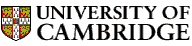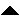Computer LaboratoryComputer Science Syllabus - Introduction to AlgorithmsIntroduction to AlgorithmsNext: Mathematics for Computation Theory Up: Michaelmas Term 2005: Part Previous: Group Project   Contents

## Introduction to Algorithms

Lecturer: Dr F.M. Stajano

No. of lectures: 4

Aims

This course is a brief introduction to discrete mathematics. It covers core elements of the subject to make the Data Structures and Algorithms course accessible to people who have not seen any significant discrete mathematics before. In particular, it covers topics which are covered in Part IA which are useful for the Data Structures and Algorithms course.

Lectures

• Common mathematical notation.

• Sets, tuples, functions.

• Relations (e.g. telephone directory) and Graphs (e.g. air routes). Induction.

• Combinations and permutations.

• O(f) notation, sorting as example.

Objectives

At the end of the course students should

• be familiar with the mathematical notation used in several areas of discrete mathematics

• be able to find O(f) notation representations of the growth rates of functions defined by certain recurrence relations

• be able to construct mathematical proofs involving induction

• be familiar with set notation and how to reason about sets and relations over sets

* Cormen, T.H., Leiserson, C.D., Rivest, R.L. & Stein, C. (2001). Introduction to Algorithms. MIT Press (2nd ed.). ISBN 0-262-53196-8
This is the recommended textbook for the Data Structures and Algorithms course, and contains enough relevant material in appendices B and C and in chapter 1 to support this short course.

Lipschutz, S. (1997). Schaum's outline of theory and problems of discrete mathematics. McGraw-Hill (2nd ed.). ISBN 0-07-038045-7
This has a good ``solved problems'' approach which may be useful for students who have not seen discrete mathematics before.

Graham, R.L., Knuth, D.E. & Patashnik, O. (1994). Concrete mathematics: a foundation for computer science. Addison-Wesley (2nd ed.). ISBN 0-201-55802-5.
This is a hardcore reference text for those who would really like to know much more about discrete mathematics.Next: Mathematics for Computation Theory Up: Michaelmas Term 2005: Part Previous: Group Project   Contents
Christine Northeast
Sun Sep 11 15:46:50 BST 2005© 2005 University of Cambridge Computer Laboratory Please send any comments to pagemaster@cl.cam.ac.uk Page last updated on 11-Sep-2005 at 15:57 by Christine Northeast